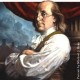## Peirce’s 1870 “Logic Of Relatives” • Comment 11.17

I think the reader is beginning to get an inkling of the crucial importance of the “number of” function in Peirce’s way of looking at logic. Among other things it is one of the planks in the bridge from logic to the theories of probability, statistics, and information, in which setting logic forms but a limiting case at one scenic turnout on the expanding vista. It is, as a matter of necessity and a matter of fact, practically speaking at any rate, one way that Peirce forges a link between the eternal, logical, or rational realm and the secular, empirical, or real domain.

With that little bit of encouragement and exhortation, let us return to the nitty gritty details of the text.

#### NOF 2

But not only do the significations of$=$  and$<$  here adopted fulfill all absolute requirements, but they have the supererogatory virtue of being very nearly the same as the common significations.  Equality is, in fact, nothing but the identity of two numbers;  numbers that are equal are those which are predicable of the same collections, just as terms that are identical are those which are predicable of the same classes.  So, to write$5 < 7$ is to say that$5$ is part of$7,$  just as to write$\mathrm{f} < \mathrm{m}$ is to say that Frenchmen are part of men.  Indeed, if$\mathrm{f} < \mathrm{m}~,$ then the number of Frenchmen is less than the number of men, and if$\mathrm{v} = \mathrm{p}~,$ then the number of Vice-Presidents is equal to the number of Presidents of the Senate;  so that the numbers may always be substituted for the terms themselves, in case no signs of operation occur in the equations or inequalities.

(Peirce, CP 3.66)

Peirce is here remarking on the principle that the measure$\mathit{v}$ on terms preserves or respects the prevailing implication, inclusion, or subsumption relations that impose an ordering on those terms. In these introductory passages, Peirce is using a single symbol$\!\!<\!\!"$ to denote the usual linear ordering on numbers, but also what amounts to the implication ordering on logical terms and the inclusion ordering on classes. Later, of course, he will introduce distinctive symbols for logical orders. The links among terms, sets, and numbers can be pursued in all directions, and Peirce has already indicated in an earlier paper how he would construct the integers from sets, that is, from the aggregate denotations of terms. I will try to get back to that another time.

We have a statement of the following form:

If$\mathrm{f} < \mathrm{m}$ then the number of Frenchmen is less than the number of men.

This goes into symbolic form as follows:$\begin{matrix} \mathrm{f} < \mathrm{m} & \Rightarrow & [\mathrm{f}] < [\mathrm{m}]. \end{matrix}$

In this setting the$\!\!<\!\!"$ on the left is a logical ordering on syntactic terms while the$\!\!<\!\!"$ on the right is an arithmetic ordering on real numbers.

The question that arises in this case is whether a map between two ordered sets is order-preserving. In order to formulate the question in more general terms, we may begin with the following set-up:

Let$X_1$ be a set with the ordering$<_1.$

Let$X_2$ be a set with the ordering$<_2.$

An order relation is typically defined by a set of axioms that determines its properties. Since we have frequent occasion to view the same set in the light of several different order relations, we often resort to explicit specifications like$(X, <_1),\$$(X, <_2),$ and so on to indicate a set with a given ordering.

A map$F : (X_1, <_1) \to (X_2, <_2)$ is order-preserving if and only if a statement of a particular form holds for all$x$ and$y$ in$(X_1, <_1),$ namely, the following:$\begin{matrix} x <_1 y & \Rightarrow & F(x) <_2 F(y). \end{matrix}$

The “number of” map$v : (S, <_1) \to (\mathbb{R}, <_2)$ has just this character, as exemplified in the case at hand:$\begin{matrix} \mathrm{f} & < & \mathrm{m} & \Rightarrow & [\mathrm{f}] & < & [\mathrm{m}] \\[6pt] \mathrm{f} & < & \mathrm{m} & \Rightarrow & v(\mathrm{f}) & < & v(\mathrm{m}) \end{matrix}$

Here, the$\!\!<\!\!"$ on the left is read as proper inclusion, in other words, subset of but not equal to, while the$\!\!<\!\!"$ on the right is read as the usual less than relation.

This entry was posted in Graph Theory, Logic, Logic of Relatives, Logical Graphs, Mathematics, Peirce, Relation Theory, Semiotics and tagged , , , , , , , . Bookmark the permalink.

### 7 Responses to Peirce’s 1870 “Logic Of Relatives” • Comment 11.17

1.Poor Richard says:

“Peirce forges a link between the eternal, logical, or rational realm and the secular, empirical, or real domain.”

There are two domains? Like “ought” and “is”? That interdigitate via links? That’s pretty remarkable if you can prove it.

•Jon Awbrey says:

These are the forms of time, which imitates eternity and revolves according to a law of number.

Plato • Timaeus

I think it it’s more like different levels of abstraction, concreteness, or detail. Different aspects, perspectives, or points from which the universe is viewed. Which raises the question, What is the geometry of the space whose points are views?

•Poor Richard says:

“Remember that time is money. He that can earn ten shillings a day by his labour, and goes abroad, or sits idle one half of that day, though he spends but sixpence during his diversion or idleness, it ought not to be reckoned the only expence; he hath really spent or thrown away five shillings besides.” ~ Ben Franklin, Advice to a Young Tradesman, 1748

This site uses Akismet to reduce spam. Learn how your comment data is processed.